# The Universe: Force and Inertia

Force may be created spontaneously by nature. However, the wilful exer­tion of a real force is also the only way a conscious being can, through the effect of inertia, cause a change of state within the physical universe and, by so doing, convey thought to another conscious being. [Português]

In the previous essay on the Notion of Motion, I considered a universe without the presence of force. The so-called forces mentioned were fictitious forces. They were not real forces. They were merely convenient conceptual props for constructing a mathematics to describe why objects in the universe seem to attract each other.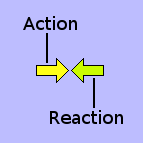Notwithstanding, real directed external force exists. Everyday experience of life on Earth demonstrates this. However, the common notion of a naked force acting in a specific dir­ection is somewhat of an over-abstraction. There is really no such thing. Forces always occur in opposing pairs or couples. As Isaac New­ton put it: "For every action, there is an equal and opposite re­action". One cannot exist without the other. There is no such thing as a physical monoforce.

A force-couple always acts like a pair of pliers. It is always compressive: never ex­pansive. When the two opposing components of a force-pair act in off-set directions (i.e. out of line), they become a torque, which is consequently opposed by an equal and opposite torque. This would suggest that an in-line (exactly-opposing) force-pair is merely a special case of a torque; namely, a torque of zero moment.The monoforce concept may, nevertheless, be useful for in­vest­igating various physical scenarios. Notwithstanding, a mono­force cannot be delivered by any physical agency. It can only be invoked within a thought experiment, within which it would have to be exerted by an imaginary agency such as the Finger of God. I shall invoke the artificial notion of a monoforce in the following thought experiment on force-induced acceleration.

In the previous article on the Notion of Motion, I showed that passive (or relative) acceleration can occur without involving a force. However, if I invoke the Finger of God to exert a monoforce on an object in free space, that object undergoes what is called active acceleration. Such active acceleration is always superimposed on top of any passive acceleration the object may have relative to any other object or observer. Thus, on the one hand, passive acceleration is always relative to another object or observer. Its value is different relative to every other object in the uni­verse. On the other hand, active — or forced — acceleration is absolute. It is "rela­tive" only to the universe as a whole.

### Force-Induced Acceleration [Linear]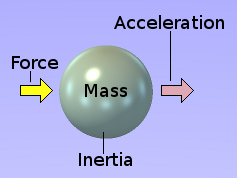In the real world, every object, such as my child­hood ball, has a property called mass. Mass im­parts to the object an effect called inertia, which is an in­herent reluctance to have its velocity cha­nged. In response to a constant external force applied to it, its inertia constrains an object to increase its velo­city at a constant rate, which is inversely propor­tional to its mass. This constant rate of change of velocity is referred to as active acceleration.

The velocity and passive acceleration of an object are, in the general sense, rela­tive to an observer. Active acceleration, however, is not. It has what could be per­ceived as an absolute frame of reference. When an external force is applied to an object for a short period, then at the end of the period, the velocity of the object will have increased relative to what it was before the force was applied.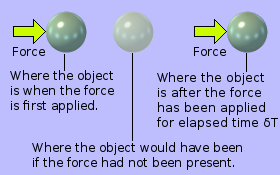The diagram on the left shows an object mov­ing right-wards within the frame of ref­erence of an arbitrary observer. The object is moving at constant velocity. A force is applied to it. It accelerates. After a period δT, the object has reached the right of the diagram. The faint image shows where it would have been, after the same period δT, if it had continued at its original velocity.

If the observer be stationed anywhere but on a collision course with the object, he perceives its "constant velocity" as a passive acceleration. It appears to undergo a non-linear deceleration up to its point of closest approach, whereupon it appears to accelerate non-linearly until it asymptotically approaches its original "constant vel­o­city". When the object accelerates under the provocation of the applied external force, this force-induced acceleration is superimposed on top of its passive accel­eration. From the point of view of the observer, the motion of the object therefore appears to be extremely non-linear and complicated.

This always necessarily places any arbitrary (relativistic) observer in a very disad­vantageous position for observing the consequences of inertia and thereby attemp­ting to understand its nature. Fortunately, this difficulty can be overcome by means of a thought experiment in which I, as the observer, commit the ultimate scientific heresy of daring to place myself in what I describe as a universal frame of refer­ence. Notwithstanding, the particular universal frame of reference to which I refer here is not of a conventional kind like that of the old luminiferous æther.

There is no universal fabric of space that imparts inertia to an object moving at con­stant velocity. Nothing fundamental in any way resists, impedes or attenuates mo­tion at constant velocity. As far as motion at constant velocity is concerned, space is empty. It consists of nothing. Plain space provides no form of linkage or coupling between separate objects within it, which may be travelling at various vel­ocities (including zero velocity) relative to each other.

Notwithstanding, as soon as an external force is applied to an object, the space through which it is passing suddenly seems to become viscous like honey or treacle. But it is a honey or treacle which only seems to resist a change in velocity. Once the externally-applied force is removed, with the object now travelling at a higher velo­city than it had initially, the viscosity of space instantly disappears, even though the object is now travelling faster through space. Thus, it seems that space becomes viscous to any accelerating object. The extent or degree to which space becomes viscous is proportional to the object's mass: not its size, as would be the case if the fabric of space were like honey or treacle.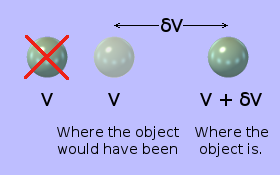The inertia of an object (the viscosity with which space resists it) is interested only in an object's change in velocity. All other objects, their relative velocities and their frames of ref­erence are irrelevant. The only frame of ref­er­ence, which is relevant to inertia, is that of the object itself. Consequently, the original posi­tion of the object, from the point of view of any arbitrary observer, is also irrelevant.

So, when an object in free space has had a constant external force applied to it for a certain time, δT, how has the object's relationship with the spatial universe chan­ged? Its change is nothing to do with distance (or separation), or even velocity. It is only to do with the object's change in velocity δV. Even the distance between where the object is now and where it would have been now if it had not been accelerated is irrelevant. This distance is, after all, constantly increasing. There exi­sts a relative velocity between where the object is now and where it would have been now.

Before the acceleration, all other objects in the universe had a certain set of velo­cities relative to the object concerned. But this was a relaxed state. The universe was not undergoing a disturbance. After the acceleration, all other objects in the universe have a different set of velocities relative to the object concerned. But this too is a relaxed state. The universe is not undergoing a disturbance. The only time the universe was undergoing a disturbance was during the acceleration of the obj­ect concerned because it was this — and only this — to which the universe reacted.

It seems, therefore, that an object's inertia is to do with that object's personal rela­tionship with the universe as a whole. If the object accelerates, the universe reacts to it, to an extent that is proportional to its mass. But if the object is moving with a constant velocity relative to all other objects in the universe, the universe does not react to it. It is completely alone. It has no connection (or coupling) with the rest of the universe.From this, I surmise that the fundamental fabric of the uni­verse must inhabit what I shall call velocity space. Thus, in its inter­action with the fabric of the universe, an object is like the arch­etypal alien warrior of science fiction with a high-technology cloaking device. So long as he keeps still, he's cloaked. You can't see him. Even if he is moving at constant velocity, you still can't see him. But if he suddenly acceler­ates, he disturbs the fabric of velocity space and thereby be­comes fleetingly vis­ible. For the human mind, formed solely from experiences with­in the terrestrial environment, the notion of acceleration does not appear to be very basic. To us, distance (or length) seems to be the most fundamental concept, followed by velocity. To the average human mind, acceleration seems to be a far more complicated concept built upon the more basic notions of dist­ance and time.

To understand inertia conceptually, I find it necessary to re-program my mind to think of the universe in a way that is somewhat different from that acquired from my experience as a planet-dweller. Instead of thinking of distance as my basic con­cept, with velocity and acceleration as its first and second differentials with respect to time, I need to think of velocity as the basic concept, and distance as its first integral with respect to time and acceleration as its first differential with respect to time. So my basic system of dimensioning becomes L=VT and A=dV/dT, where A is acceleration, V is velocity and L is length (or distance).

So, just as a [dimensionless] point is a position in point-space, so a velocity is a [dim­ensionless] position in velocity space. An observer in velocity space thus, like the Beatles' Nowhere Man, "doesn't have a point of view": he has a "velocity of view" — as cumbersome as that may sound. As a length in point-space is the dist­ance be­tween two points, so a "length" in velocity-space is the difference between two velo­cities: in other words, the relative velocity between an object before accel­eration and the velocity of the same object after acceleration.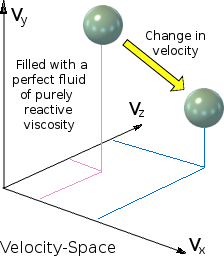Objects in space, moving at different velocities relat­ive to each other, all seem to be stresslessly attached to the same universal fabric. This makes it a very str­ange kind of "fabric" for us Earth-based folk to visual­ise. If such an object undergoes a short force-induced acceleration, it changes to a different velocity, repres­ented by a different posi­tion in velocity space. The old and new velocities are irrelevant. Only the change in velocity is relevant. Consequently, the origin of the coordinate axes, as shown on the right, is also irrele­vant. This change in velocity can only be accompl­ished by the object accelerating. This stresses the universal fabric, which reacts with equal and op­posite inertial resistance.

Thinking under this new conceptual orientation, it seems that this fundamental uni­versal fabric only manifests its "substance" to an object that is accelerating. It is as if velocity-space, as illustrated in the graph above, were filled with — or even itself comprised — a viscous fluid. Thus, if an object accelerates, it moves (changes its location) within velocity-space and thereby encounters the resistance of this visc­ous fluid.

Consequently, differentiating velocity with respect to time somehow creates a cou­pling with the fundamental fabric of the universe. Integrating velocity with respect to time somehow creates the condition for independent objects to integrate into what may be perceived as static structures. And it is from these that all that we know — including ourselves — are made. And these all — including ourselves — have the attr­ibute we call inertia. Thus, inertia is the unique means by which we each may react directly with the fundamental fabric of the universe.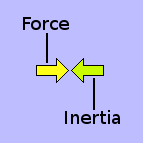The significance of all this is that the monoforce exerted, within my thought experiment by the Finger of God, is always opposed by an equal and opposite "force" of inertia. Notwithstanding, the "force" of inertial reaction isn't applied to the oppo­site side of the object's surface. It seems to be applied at every point within the substance of the object. It is as if every indivisible point of substance within the object is being in­dependently "resisted" by the "viscosity" of velocity-space.

### The Energy Problem

If I accelerate, I disturb the oceanic fabric of the universe. This requires that an ext­ernal force be applied to me for a certain amount of time, which supposedly would consume energy. Where would this energy go?

Some would say that it becomes an increase in my kinetic energy. But an increase in my kinetic energy is relative to other objects in space, according to by how much their various velocities have increased relative to me. My acceleration could equally well be regarded as a deceleration relative to yet other objects in space, in which case, my kinetic energy will have decreased relative to them. Kinetic energy is not therefore an intrinsic property of an object. It is an intrinsic property of the relation­ship between two objects. This makes sense because kinetic energy is a function of velocity, which itself is an intrinsic property of the relationship between two obj­ects.If I apply a force to a mass (moving at velocity V) for a time δT, as shown on the right, it will accel­erate up to a higher velocity (V+δV) in the direc­tion of the force. The mass's kinetic energy will have increased by ½M(δV)2. If I now apply the same amount of force to the mass for the same amount of time in the opposite direction, the mass will revert to its ori­ginal velocity V. And its kinetic energy will be back where it started.

The mass's overall kinetic energy, relative to all other objects in the universe, has not changed. Notwithstanding, the externally applied force F has supposedly exp­ended a total amount of energy M(δV)2. Where has this energy gone? The applied external force was resisted by the mass's inertia. Its inertia is an absolute quantity, which is independent of, and nothing to do with, other objects in its spatial vicinity.

My body has a mass of 82 kilograms. Suppose I am in free space. My rocket-pack applies an external force of 804 newtons to my body. Neglecting the mass of my space-suit and rocket-pack, my body will thus accelerate at 9·80665 metres per second per second. Suppose I turn off my rocket-pack when I have been accelera­ting for one kilometre. The rocket-pack will have delivered 804000 newton-metres (or joules) of useful energy. That is equivalent to 223·33333351 watt-hours, which is about 0·223kWh (standard domestic unit in which electricity is sold).

I am not a very big object. A rocket-pack able to deliver 804 newtons of thrust isn't all that powerful as rocket motors go. An amount of energy approaching ¼ of a kilowatt-hour is an amount of energy that is well within the bounds of scientific instr­umentation to measure, even if its energy density diminishes as the inverse square of the measuring instrument's distance away from me in my rocket-suit.The energy dissipated by my inertia in "resisting" the applied external force must therefore have been absorbed by some­thing absolute; namely, the oceanic fabric of the uni­verse. If true, this would necessitate that the energy must have been transferred from me, via my inertia, into some kind of wave-like disturbance within the universal fabric. As such, it would then have to travel outwards away from me through the univ­ersal fabric in the form of an ever expand­ing spherical shell.

Yet no such kind of energy-wave is detectable. The amount of energy delivered by my rocket-pack is what could be called absolute energy. It was energy that lay capt­ive in chemical bonds. When it was released, it created a relative dynamic energy between me and zillions of gas molecules that the rocket ejected in the opposite direction to the way it pushed me. The released chemical energy all went into spreading these gas molecules in space, thus increasing the amount of disorder (or entropy) in the universe. But none of the energy delivered by my rocket-pack seemed to go towards the task of overcoming the resistance offered by my inertia. My inertia exerted an equal and opposite force to that exerted by the rocket. But it was a force that did not seem to dissipate energy.It seems, therefore, that the universal fundamental fluid that I sup­pose must fill velocity-space, has what appears to be a non-dissip­ative viscosity. Consequently, I surmise that inertia be not a resist­ance to acceleration but a reactance to it. The force and the move­ment must be somehow out of phase, in the way that vol­tage and current are in an electrical circuit that is purely reactive. This univ­ersal reactively-viscous velocity-æther somehow ind­uces what may be thought of as an inertial-charge into any material that is acceler­ating through it.

The principle that the velocity-æther is disturbed by an accelerating object has an important consequence. Suppose that a momentary accelerating force (a pulse) is intentionally applied to an object by a conscious being. The object accelerates. In so doing, it disturbs the velocity-æther, which reacts in what appears as inertia im­parted to the accelerating object. The ætherial disturbance then travels outwards in all directions on a journey that will eventually take it to the furthest reaches of the universe.

If, at some distance from the original object, another object is able to transduce that ætherial disturbance back into a pulse, then a communication sys­tem exists. Thus, a pulse, wilfully effected by one conscious being, can be used to convey a basic unit of information [a bit] to another conscious being.

Notwithstanding, there is no evidence that other individual objects in the universe are affected in any way by such an 'inertial wave', whose energy content — and hence effect — ought to be quite strong. So where can this energy have gone?

### Force-Induced Acceleration [Orbital]

The common notion is that each observer has a unique frame of reference, which is velocity-based and which is relative to the velocity-based frames of reference of all other observers and objects of observation. No particular observer can claim to be stationary in any absolute sense. But is there such a thing as a universal frame of reference, which is common to all observers and objects of observation? At first sight, there seems to be. It is the rotational frame of reference, whose basis of measurement is not linear velocity but angular velocity.The ballet dancer on the right can sense that she is rotating be­cause the extremities of her body (her arms and right leg) feel a centrifugal force pulling them outwards. The strength of this force is proportional to the square of her angular velocity. If she pulls-in her arms and right leg towards her centre of rotation, she acceler­ates rotationally. If she then allows her arms and right leg to be taken outwards again by the centrifugal force, she deceler­ates rot­ationally to her original angular velocity.

If she feels no centrifugal force at all acting on her limbs, she can correctly claim, independently of seeing anything, to be absolutely not rotating. However, it is not quite that simple.Imagine that the two spheres in the outer orbit on the left are linked by a weightless thread. A dæmon†, at the orbital centre, now pulls equally on both halves of the thread, forcing the spheres to the inner orbit. If they were orbiting freely at the inner radius, they would revolve faster than in the outer orbit anyway. However, the force, exerted by the dæmon, caused the spheres to accelerate rotationally, independently of any gravitation­al considerations. Consequently, the spheres now revol­ve much faster than they would do if they were simply in free orbit at the inner radius.

† A dæmon is an imaginary intelligent being who has no mass, occupies no space, and is capable of exerting a reactionless force on a physical ob­ject. His quest is to use his nature and ability, within my thought experi­ments, to help me illustrate what I am trying to explain.

In the current situation, the thread is tensed by a centripetal force, F. Now suppose the dæmon releases his hold on the thread so that it can extend to its full length again. The two spheres move outwards to the outer orbit where they started. The thread is now slack again and the spheres adopt a free orbit around their common centre. They suffer no centripetal force. No energy is expended during this process (apart from a trivial amount to release the thread from the spheres, which is not relevant to the process).

The spheres are now back exactly where they were before. The energy state, be­fore and after the dæmon did his thing, is the same. Notwithstanding, in hauling in the spheres to the lower orbit, the dæmon did some work. He expended energy. If energy be conserved, where did it go?

### The Energy ProblemNow let's try something else. It requires two dæmons. The dæmons each go to a different one of the two sph­eres revolving in the free outer orbit. Each then pushes his respective sphere in the opposite direction to that in which the sphere is revolving around the common cent­re. The dæmons apply equal force to their respective spheres for exactly the same amount of time. This caus­es the spheres to fall to the inner orbit. However, in this case, the inner orbit is now a free orbit. The spheres are not joined by a thread and therefore are not experien­cing any centripetal force.

The dæmons each exerted a force over a certain distance. Consequently, each exp­ended energy in order to transfer his sphere from the outer free orbit to the inner free orbit.Now in the inner orbit, each dæmon pushes his resp­ec­tive sphere in the direction in which it is revolving ar­ound the common centre. Each applies the same am­ount of force for the same amount of time. The spheres move back to the outer orbit. Energy was again expen­d­ed by the dæmons in order to transfer the spheres from the inner free orbit back to the outer free orbit. Thus, energy is required to transfer the spheres from one free orbit to the other. This is true whether the orbital radius is being increased or decreased.

Thus, a wider orbit does not necessarily signify a higher energy.

This can be a little bit counter-intuitive for Earth-dwellers. When a weight is raised upwards here on Earth, it is said to gain potential energy. This is recovered when the weight falls back again to its original height, as in the case of a weight-powered clock. But an object in orbit does not have in­herent potential energy. It would only do so if it were not revolving. To stop an orbiting object revolving requires the expenditure of energy. This expended energy would then be its potential energy relative to what it had been orbiting around.

So what happens to the energy that the dæmons expended each time? If energy be conserved, where does it go?

It would seem that absolute zero rotation — the absence of a centripetal force — is when you are going round in a stable free orbit: not when you are simply not going round. In different stable orbits, you go round at different rates. So the rotational frames of reference of different observers are different.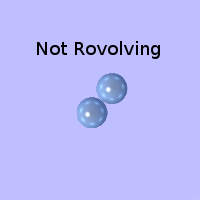Suppose the dæmons keep applying a force to their re­spective spheres, against the direction of orbit, until the orbit of the two spheres has reduced to zero. The sph­er­es are simply resting against each other, the common centre of orbit being the point at which they touch each other. The dæmons fine-tune the situation so that they reach the point at which the surface of each sphere pushes against the other with maximum reaction to what is conventionally viewed as their mutual gravita­tional attraction. That is, the "weight" of each sphere up on the other is at its maximum.

This would seem to be some kind of absolute zero point within a universal frame of reference. It is intuitively evident that this zero-point for absolute rotation should be the same for any and all systems of revolving or rotating objects. It seems therefore to be a frame of reference whose zero-point is relative to the universe as a whole.

A physical corollary of this is that the relationship between an observer and an obj­ect he is observing cannot be one of simple relative velocity within a Cartesian frame of reference. Both the observer and the observed must necessarily be revol­ving within their respective orbits — however long, meandering or complex — with­in the universe as a whole. Consequently, if revolution were to be removed from the universe, all mass would rapidly "fall" to a single location. The universe would thus become the one and only super black hole.

Now, what about the disappearing energy? Where does the energy go that is requ­ired to both increase the radius of a sphere's free orbit and also to reduce it? The monoforce, which each dæmon applies to his respective sphere is opposed by an equal and opposite inertial reaction.

The energy expended by a force, acting upon an object in space, is independent of whether the object is way out in free space, beyond the sight or influence of any other objects, or whether it is in orbit around another object close by. Consequent­ly, the energy dissipated by the sphere's inertia in opposing the force, must be absorb­ed by something absolute; namely, the fundamental fabric of the universe.

If true, this would necessitate that the energy must have been transferred from the sphere, via its inertia, into some kind of wave-like disturbance within the universal fabric. As such, it would then have to travel outwards away from the sphere, thro­ugh the universal fabric, as a wave in the form of an ever-expanding spherical shell.

The upshot, of all that has so far been discussed herein, is that inertia, rather than being an inherent property of matter, must be merely an induced property, which only becomes manifest when the matter is accelerating relative to the universal fabric: the velocity-æther.

### Force Without Acceleration

I have previously discussed the case of an object in free-fall. In free-fall, no force is acting on what is clearly an accelerating body. On the other hand, when an object is resting on the ground (on the surface of a planet), it is being acted on by a force (its weight). But it does not accelerate. It doesn't even move at constant velocity. It simply stays put.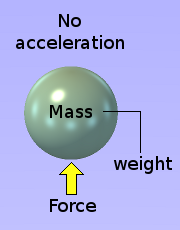The surface of a planet exerts an upward force upon any mass that is resting on it. I am standing on the surface of the Earth. My body has a mass of 82 kilograms. So the ground is pushing on the bottoms of my feet with a force of 804 newtons. Consequently, I should be accelerating up­wards at 9·80665 metres per second per second, which is the acceleration of the Earth's gravity, so-called. I live on the surface of the Earth. So as each second of my life passes, the upward velocity of my body must increase by 9·80665 metres per second. The only limitation on my final velocity would appear to be nothing other than the velocity of light, as decreed by the theory of relativity.

The speed of light is said to be 299792458 metres per second. By standing on the Earth's surface, I will therefore attain the speed of light in 299792458 ÷ 9·80665 = 30570322·995110461 seconds. That is 30570322·995110461 ÷ (60 × 60 × 24) = 353·823182814 days. That's just about 12 days short of a year.

Notwithstanding, I have currently been on the Earth's surface for nearly 72 years and I am still here. I am not hurtling away from the Earth at 70 times the speed of light (or at almost the speed of light while exhibiting many times my rest mass). If acceleration be defined as the rate of change of velocity and velocity be defined as the rate of increase in the distance between two objects then, when I am standing on the surface of the Earth, I am clearly not accelerating. Thus a situation exists in which an external force, acting upon a mass, does not cause that mass to acceler­ate.

The force I feel on the bottoms of my feet when I am standing on the surface of the Earth is called my weight. My weight is an external force, which does not cause my mass to accelerate. Furthermore, my weight is not simply an applied force of arbit­rary magnitude, as would be a force applied to a mass in free space that causes it to accelerate. The magnitude of my weight depends on the magnitude of my mass. So, what appears to be acting upon me when I am standing on the ground is not simply a force but a force per unit mass. In the case of anything resting on the sur­face of the earth, this amounts to 9·80665 newtons per kilogram.An external force, applied in line with the centre of mass of an object in free space, causes that object to acceler­ate in a straight line — no matter what shape that object may be or how large a volume it may occupy. Weight, however, does not apply force in the same direction to all parts of a mass resting on the Earth's surface. Weight is spherically divergent. For instance, if we consider the South American contin­ent, the "weight-force" acting up­wards on the under­side of Patagônia is acting in a differ­ent direction than the "weight-force" acting upwards on the underside of Venezuela.

A force that causes a mass to accelerate is linear and has a magnitude which is independent of the magnitude of the mass it is accelerating. Weight, on the other hand, is a spherically-divergent field of force per unit mass, which produces no acceleration. If the force of the weight of an object were to cause it to accelerate, it would tend to spread the object as it went, especially if the object comprised lots of uncoagulated granules. But this does not happen because the object is simply not accelerating. Inertial force and weight are related to mass. But they are not the same thing. They are not equivalent. They are conceptually very different. No con­ceptual connection has, as far as I know, ever been officially established between them.

### Back On The Ground

With my feet back on the ground, I feel a real tangible force acting upwards on the bottoms of my feet, yet clearly perceive that I am not accelerating in any direction. I am not getting further and further away from where I am with ever-increasing haste. But the force I feel should, according to the observed law: force = mass × acceleration, be accelerating me. So perhaps I am accelerating. I must look more closely at the relationship: force = mass × acceleration.

If I am a body in free space and a steady external force is applied to me, I acceler­ate linearly. While I am accelerating, the force acting upon me is in exact equi­librium with the reactionary force of my inertia. This inertial force is proportional to my mass. My mass is some kind of notion of the amount of substance in my body. It is as if my substance is connected to a velocity-fabric (or velocity-æther), which is reluctant to be distorted by my changing velocity (my acceleration).

Thus, in free space, there is a tangible relationship between the substance of my body and the velocity-æther. My body accelerates: the velocity-æther resists the force that is causing me to accelerate. So, perhaps too, on the ground, something is accelerating while something else, to which it is related, is, in the same way, resist­ing that acceleration. To the human senses, with which nature has equipped me, I am unaware of anything relative to which I could be accelerating. So, if indeed I am accelerating relative to something, it must be something, which nature has seen no need for me to have to be aware of.

Notwithstanding, nature has furnished me with deductive reasoning. And this leads me firmly to suppose that what is accelerating relative to me — and indeed the rest of all that I see — must be the universal velocity-æther itself. It is forever acceler­ating downwards into the ground like a giant 3-dimensional waterfall.As I have said, my body weighs 82 kilograms. The ground is thus pushing on the bottoms of my feet with a force of 804 newtons. Consequently, I must be accelerating at 9·80665 metres per second per second in an upward direction. Not­withstanding, this acceleration, contrary to nat­ural intuition, is not relative to the Earth nor to anything on or beyond the Earth. The upward force of 804 newtons is causing me to ac­celerate upwards relative to the supposedly downward flow­ing æther. Rem­ember, however, that this intangible universal æther is a velocity æther; not a static one. It only manifests itself to accelerating substance. Con­sequently, at the Earth's surface, the æther is not acceler­ating at 9·80665 metres per second per second but is merely flowing at a const­ant velo­city, c.

But where is it going? It is going into the Earth. But why? My best suggestion is that somehow the Earth is continually sucking in this universal velocity-æther.

If I were standing on the Moon, my body would still have the same mass of 82 kilo­grams. However, my weight — the upward force, which the surface of the Moon would be exerting on the bottoms of my feet — would not be 804 newtons. It would be only just over 133 newtons. It would therefore be as if I were accelerating only at 1·6249 metres per second per second. Thus, although the velocity-æther is flow­ing into the Moon's surface at the same velocity, namely c, its density, ρ, is only 0·165422886 what it is at the Earth's surface because the Moon contains much less mass than the Earth.

It would thus appear that what determines the rate at which the velocity-æther is being sucked in is the amount of mass. So it is perhaps reasonable, at this stage, to deduce that mass sucks in the velocity-æther, causing it to continually flow into a material object. And in the case of me standing on the Earth, it must do so in a manner that makes the æther's density inversely proportional to the square of the distance between the centres of my mass and the mass of the Earth.

### The Myth of Mass

The foregoing implies that the force, which the ground is exerting on the bottoms of my feet, be merely a reaction to an inertial force (my weight), which is induced within my body by the downwardly-flowing velocity-æther. Thus, the force, which the ground is exerting on the bottoms of my feet, is merely cancelling the induced inertial force that is attempting to accelerate my body downwards into the Earth, thereby keeping me exactly where I am on the Earth's surface.Hereby is established a relationship — an equivalence — betw­een weight and inertia. But where does this leave the notion of mass? Mass is not a directly observable quantity but it is pre­sumed to be the underlying fundament of both weight and in­ertia, which are both directly observable quantities. Hence, if weight (a gravitational force) be the same thing as inertia (a dynamical force), and inertia be an induced rather than an intrinsic property of matter, then the notion of mass, as an intr­insic property of matter, becomes a non-entity. Matter is intrin­sically massless. Mass was never any­thing more than a con­ceptual aid anyway. This makes one of the most stalwart pillars of the mass-length-time (MLT) system of dimensions no more than an illusion.

The notion that matter be inherently massless begins to shed light on previously ir­reconcilable experimental observations in which substantial macroscopic objects, such as Professor Eric Laithwaite's precessing flywheels, appear, in some circum­st­ances, to "lose" both weight and inertia. This is reconcilable with the observation that mass is relativistic: it varies with relative velocity. Consequently, mass cannot be an inherent property of an object. It has to be a property of the dynamic rela­tionship between an object and a specific observer who is observing it at a partic­ular time.The notion, that matter is intrinsically massless, is also in harmony with observation and theory at the micro­sc­opic scale. According to the Standard Model, the primary particles should all be massless. So perhaps the appar­ent mass, which most of them have, is an in­ertial react­ion induced within them by the universal velocity-æther as they accelerate and decelerate rel­ative to it. This further alludes to the idea that the universal velocity-æther, which I have proposed in this essay, could be that elusive necessity which physicists call the Higgs Field.

© 19 May 2013, 05 May 2016 Robert John Morton | PREV | NEXT